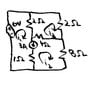# Solving Mesh Analysis Equations for i1, i2 and i3

• vs55

## Homework Statement

Use mesh analysis to determine i1,i2 and i3

## The Attempt at a Solution

using mesh analysis i get rid of the 3A current source and the 1ohm resistor
1) -6 + 2(i1 - i3) + 4(i2 - i3) + 8i2 = 0
2) 2(i3 -i1) + 2i3 + 4(i3 - i2) =0
3)i1 + i3 = 3

i'm not sure if i have the right equations set up. For the last equation i also used
i1 + i3 = 3 +i2 to try and solve but the answer was still wrong
i have no idea what i have wrong

#### Attachments

•Untitled.jpg
19.3 KB · Views: 383